# How To Solve Venn Diagrams Questions Quickly

## How To Solve Venn Diagrams Questions

• A Venn diagram is an illustration of common characteristics.
• Maths logic a diagram in which mathematical sets or terms of categorical statement are represented by overlapping circles within a boundary representing the universal set, so that all possible combinations of the relevant properties are represented by various distinct areas in picture.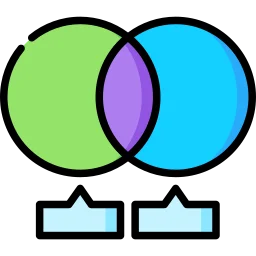### How To Solve Quickly Venn Diagram

Question 1 How many numbers are there between 1 and 100 that are not divisible by 2, 3 and 5 ?

Options

(a) 24                     (b) 20

(c) 25                     (d) 22

Solution:-  From the diagram it is clear that

(27+14+7+7+13+3+3 = 76 ) 76 numbers are divisible by either 2,3 or 5.

So 100 – 76 = 24 numbers are not divisible by 2,3 or 5.

Correct Option : (a)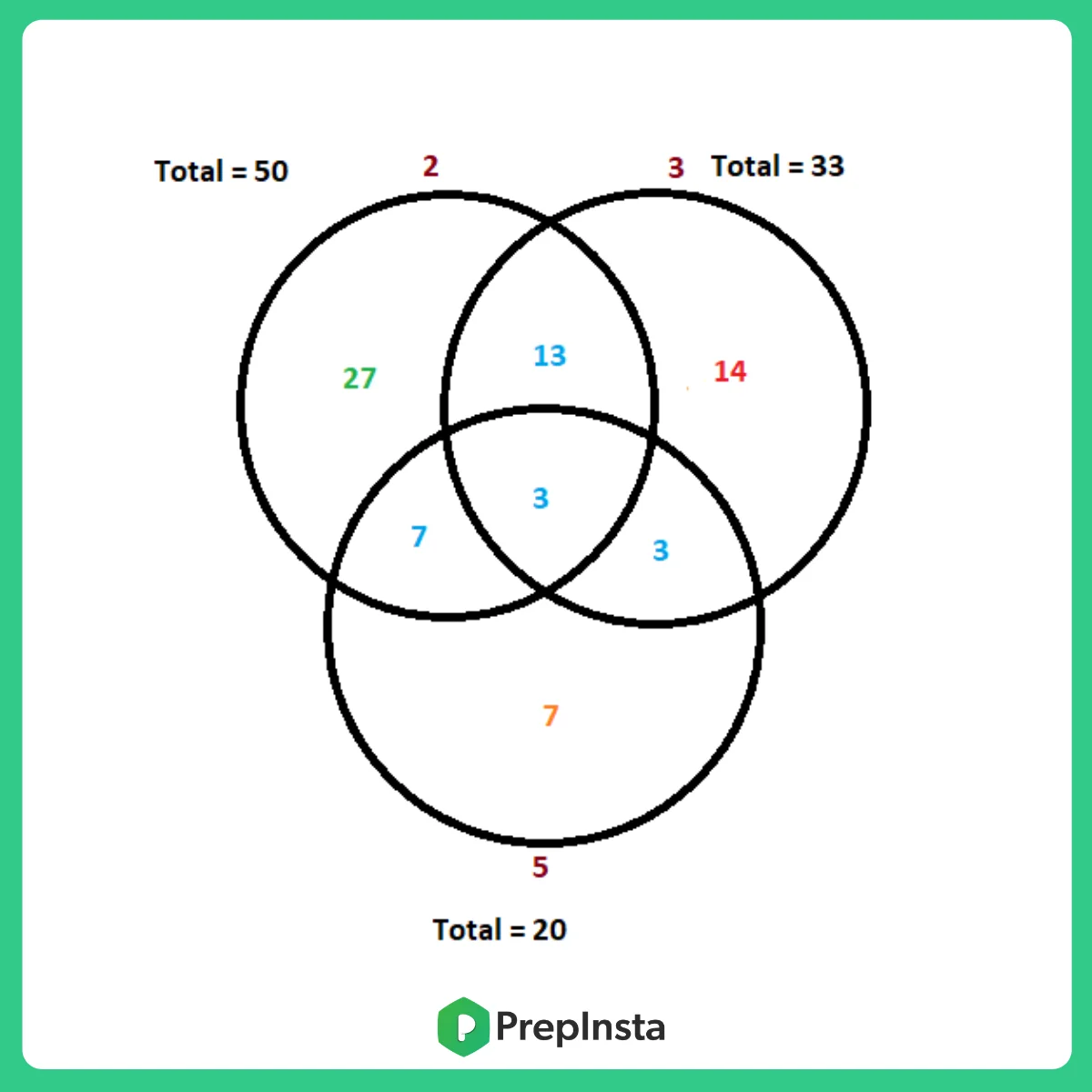Questions 2 How many students study only one of the three subjects?

Options

(a) 21                     (b) 30

(c) 39                    (d) 42

Solution:-  From the above diagram, you can clearly see that 3 students study all three subjects.

Number of students who study only one subject = 18 + 9 +12 = 39.

Correct Option : (c)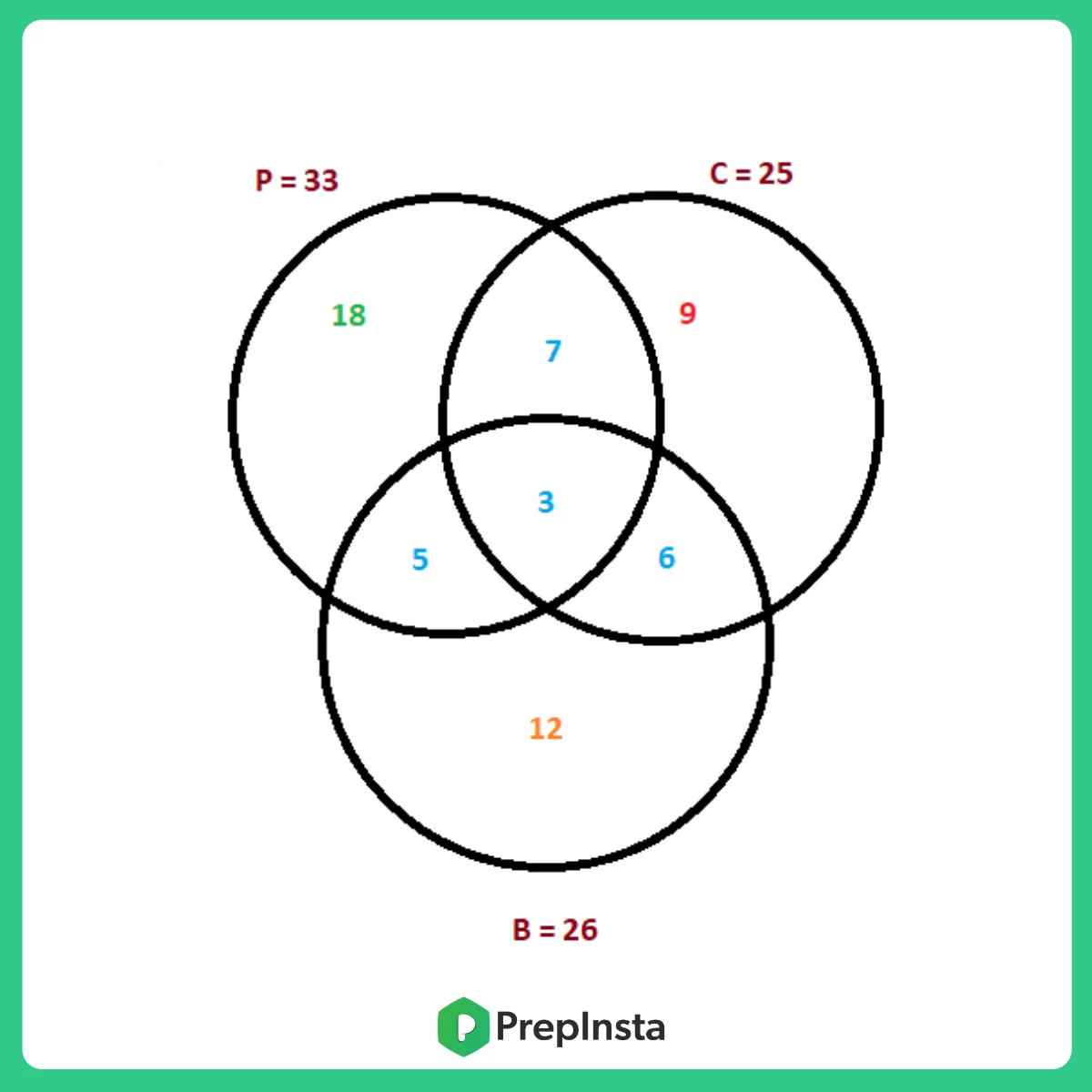### Related Banners

Get PrepInsta Prime & get Access to all 200+ courses offered by PrepInsta in One Subscription

Question 3 In the following diagram, triangle represents people who can sing, circle represents people who can paint. If the number of people who know to do exactly 2 of the above mentioned things is equal to the number of people who can paint is

Options

(a) 23                      (b) 13

(c) 53                       (d) 63

Solution :

Number of people who can do exactly 2 out of the 3 activities = 12 + 21 + 20 = 53.

Let the missing value be ‘x’.

Number of people who can do exactly one of the 3 activities = 23 + 7 + x = 30 + x.

It has been given that 30+x = 53

x = 23

Number of people who can paint = 12+8+20+23 = 63.

Therefore, option D is the right answer.

Correct Option: D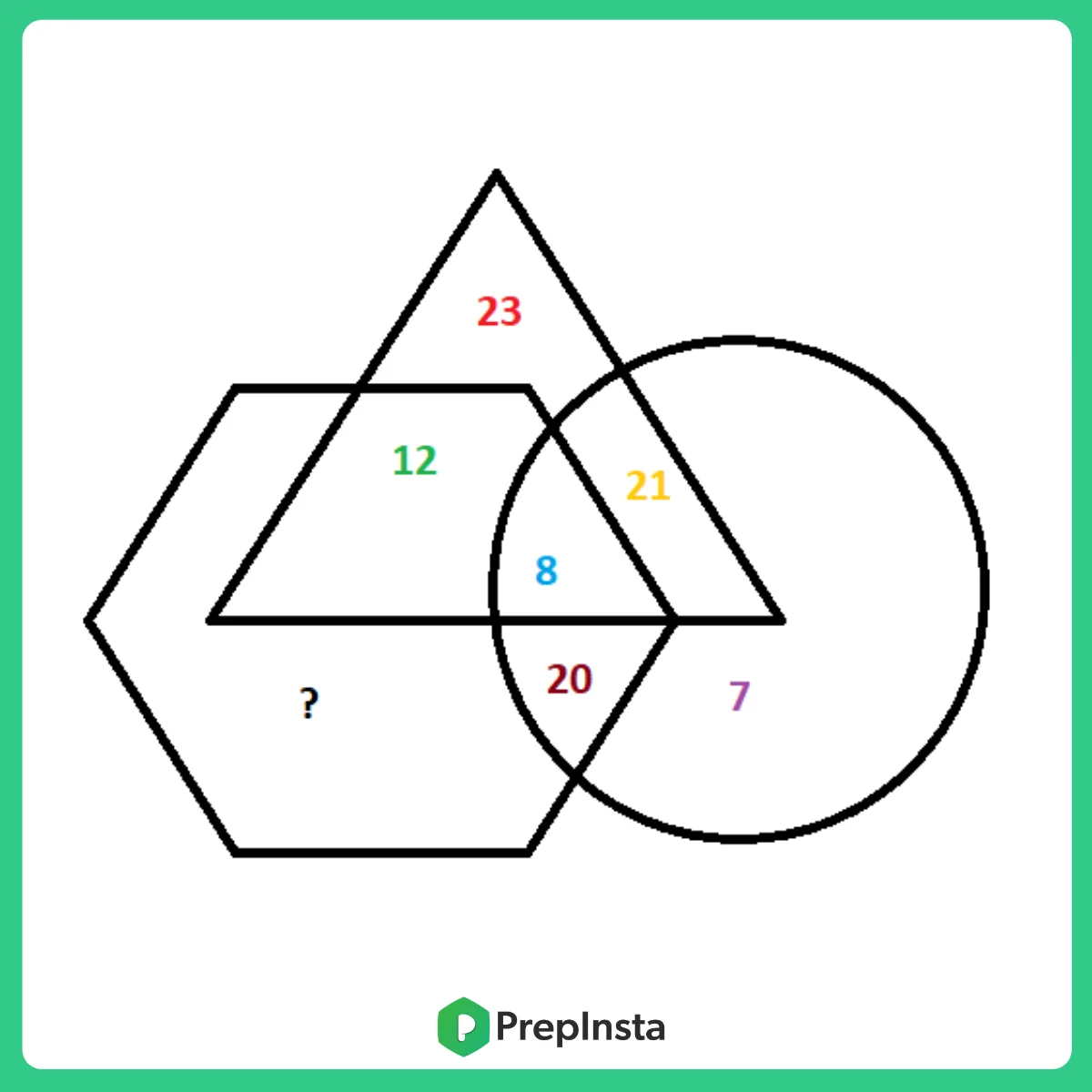Questions 4 In the following diagram, each geometric shape represents a movie

Options

(a) 5                    (b) 14

(c) 13                   (d) 9

Solution :   The area common to the circle, trapezium and the hexagon but not common to the triangle represents the people who can sing, swim and paint but cannot dance.

From the diagram, we can see that the number of such people is 14. Therefore, option B is the right answer.

Correct Option: B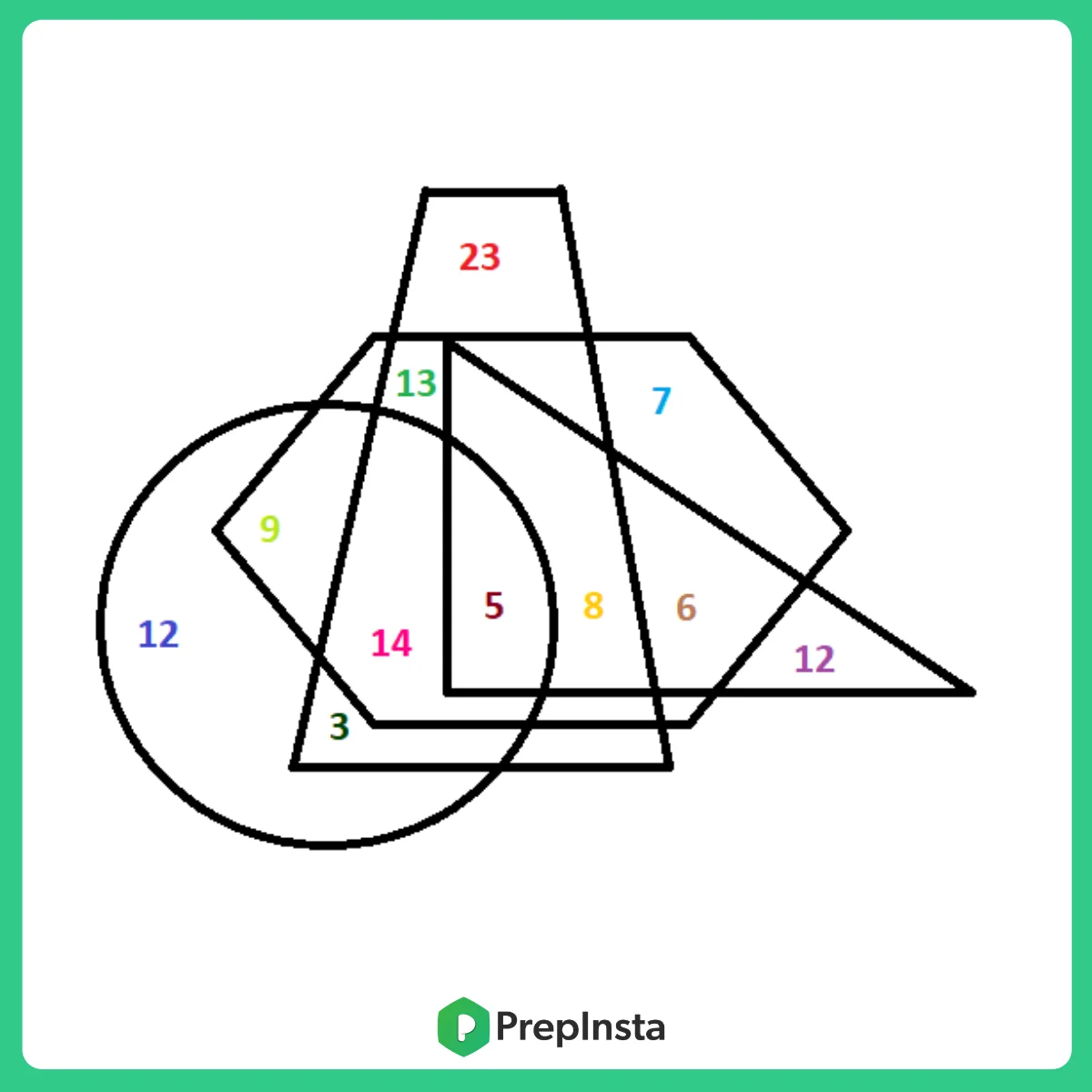Question 5: From the below given venn diagram find out the number of people who neither play cricket nor football.

Options

a)195        b)350

c)230        d)165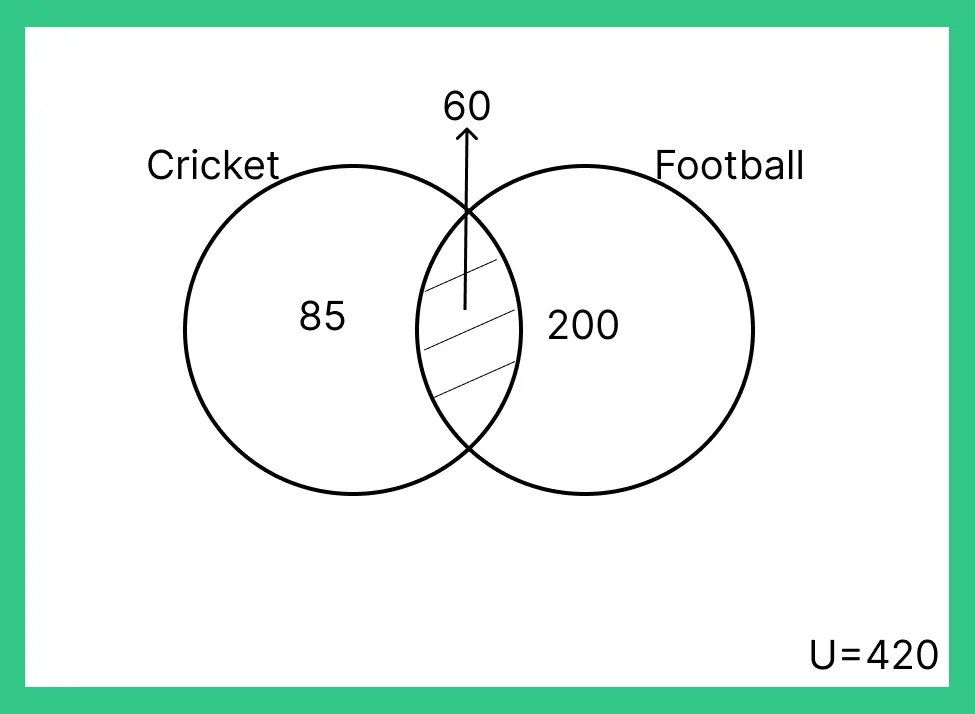Solution: Number of students who play only cricket- 80-60= 20
Number of students who play only Football- 200-60=140
Number of students who play both= 60
Number of student who play none= 420-(140+20+60)
Correct Option :A

## Get over 200+ course One Subscription

Courses like AI/ML, Cloud Computing, Ethical Hacking, C, C++, Java, Python, DSA (All Languages), Competitive Coding (All Languages), TCS, Infosys, Wipro, Amazon, DBMS, SQL and others